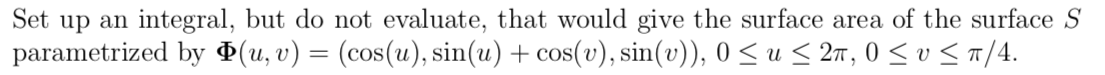# Set up an integral, but do not evaluate, that would give the surface area of the surface S parametrized by (u, v) = (cos(u), sin(u) +cos(v), sin(v)), 0 u 2T, 0 v

Question

Thank you!!!help_outlineImage TranscriptioncloseSet up an integral, but do not evaluate, that would give the surface area of the surface S parametrized by (u, v) = (cos(u), sin(u) +cos(v), sin(v)), 0 u 2T, 0 v